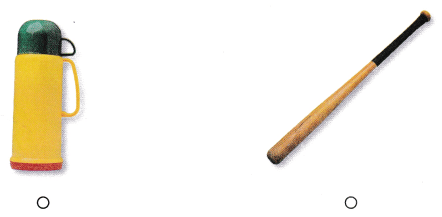# Texas Go Math Kindergarten Lesson 19.1 Answer Key Measuring an Object

Refer to our Texas Go Math Kindergarten Answer Key Pdf to score good marks in the exams. Test yourself by practicing the problems from Texas Go Math Kindergarten Lesson 19.1 Answer Key Measuring an Object.

## Texas Go Math Kindergarten Lesson 19.1 Answer Key Measuring an Object

Essential Question
How can you solve problems by drawing a picture?

ExploreDirections
Katie wants to measure the jar. What can she find out?

Share and Show

Question 1.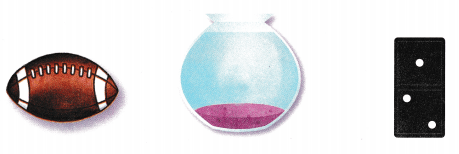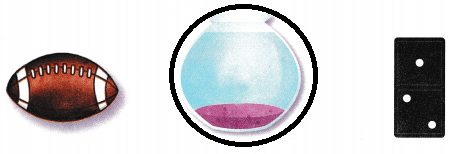Explanation:
A pot holds the water
so, circled the pot.

Question 2.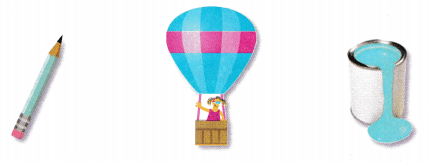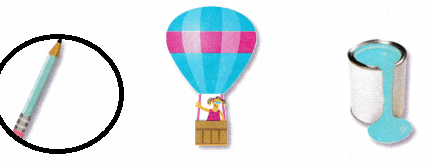Explanation:
Pencil is measured by length
so, circled the pencil.

Question 3.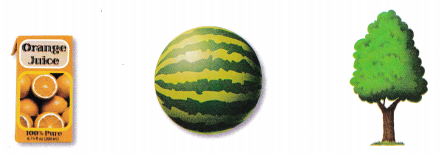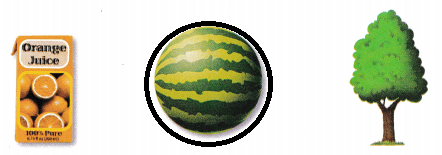Explanation:
Water melons are measured by weight
so, circled the water melon.

Directions
1. Which object would you most likely measure to find out how much it holds? Circle the object. 2. Which object would you most likely measure to find out how long it is? Circle the object. 3. Which object would you most likely measure to find out how much it weighs? Circle the object.

Question 4.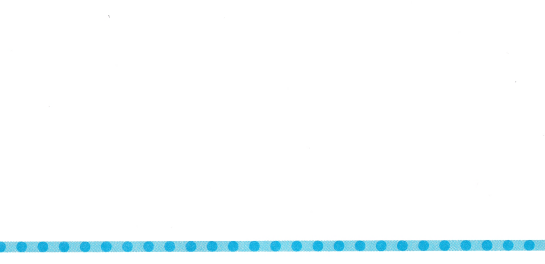Explanation:
Apples are measured by weight
so, drawn a apple.

Question 5.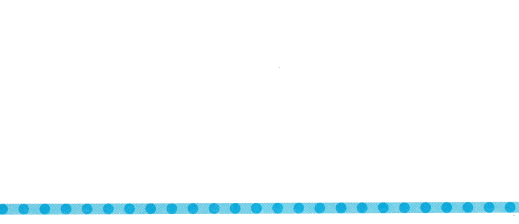Explanation:
A jar is used to find how much it holds
so, drawn a jar

Question 6.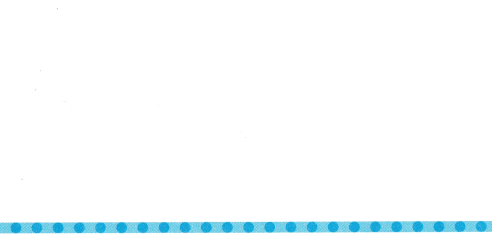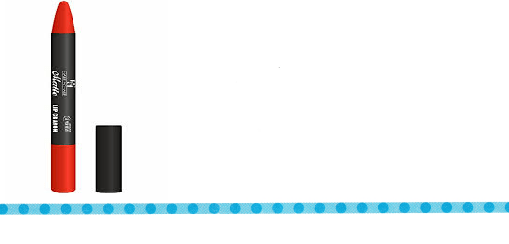Explanation:
crayon is measured by length
so, drawn a crayon.

Directions
4. Draw on object that you would measure to find its weight. 5. Draw an object that you would measure to find how much it holds. 6. Draw an object that you would measure to find its length.

Question 7.Explanation:
Vegetables are measured by weight
so, drawn the vegetables.

Question 8.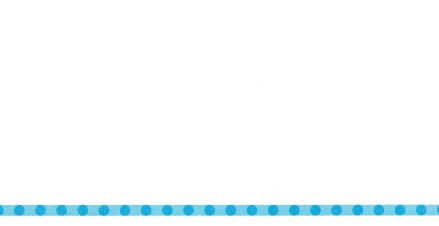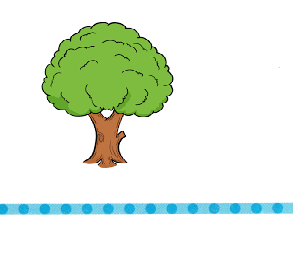Explanation:
A tree is measured in length
so, drawn a tree.

Question 9.Explanation:
A milk packet shows how much milk it holds
so, drawn a milk packet.

Directions
7. Draw an object that you would measure to find its weight. 8. Draw an object that you would measure to find its length. 9. Draw an object that you would measure to find how much it holds.

### Texas Go Math Kindergarten Lesson 19.1 Homework and Practice

Question 1.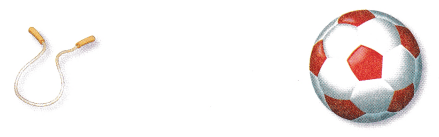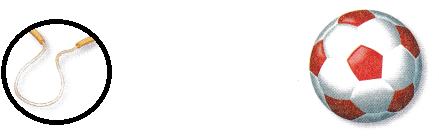Explanation:
Rope is measured in length
so, circled the rope.

Question 2.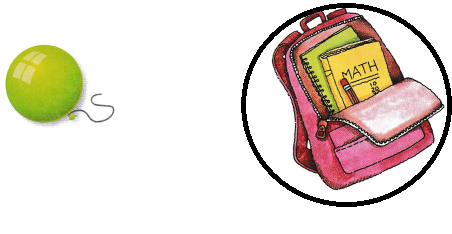Explanation:
The bag is measure in weights
so, circled the bag

Question 3.Explanation:
A jar is used to measure the liquid
so, marked the jar.

Directions
1. Circle the object you would most likely measure to find how long it is. 2. Circle the object you would most likely measure to find how much it weighs. 3. Circle the object you would most likely measure to find how much it holds.

Texas Test Prep

Question 4.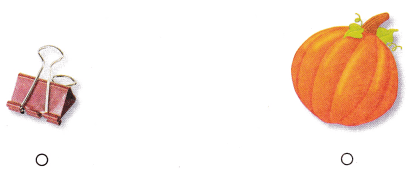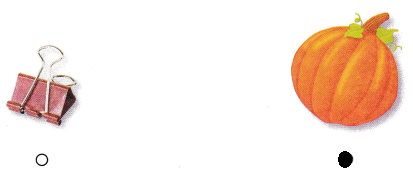Explanation:
Pumpkin is measured by weight
so, marked the pumpkin.

Question 5.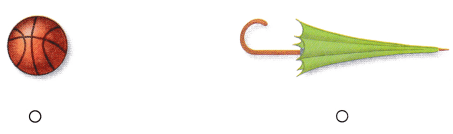Explanation:
The umbrella is used to find the length
so, marked the umbrella.

Question 6.